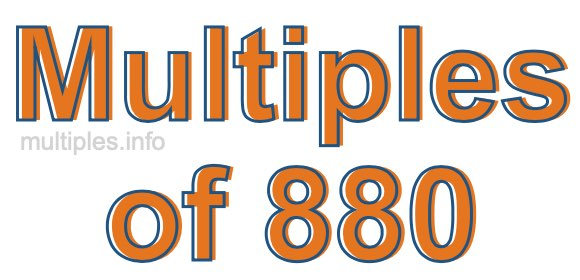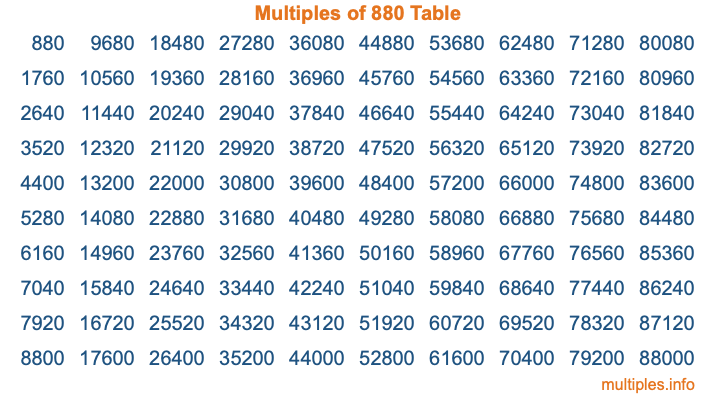Multiples of 880Welcome to the Multiples of 880 page. Here we will first teach you everything you will ever need to know about the multiples of 880, and then give you a study guide summary of everything we taught you to make sure you remember it all. Use this page to look up facts and learn information about the multiples of 880. This page will make you a multiples of eight hundred eighty expert!

Definition of Multiples of 880
Multiples of 880 are all the numbers that when divided by 880 equal an integer. Each of the multiples of 880 are called a multiple. A multiple of 880 is created by multiplying 880 by an integer.

Therefore, to create a list of multiples of 880, you start with 1 multiplied by 880, then 2 multiplied by 880, then 3 multiplied by 880, and so on for as long as you want. Thus, the list of the first five multiples of 880 is 880, 1760, 2640, 3520, and 4400. To see a larger list of multiples of 880, see the printable image of Multiples of 880 further down on this page. We also have a category where you can choose any nth multiple of 880.

Multiples of 880 Checker
The Multiples of 880 Checker below checks to see if any number of your choice is a multiple of 880. In other words, it checks to see if there is any number (integer) that when multiplied by 880 will equal your number. To do that, we divide your number by 880. If the the quotient is an integer, then your number is a multiple of 880.

Is  a multiple of 880?

Least Common Multiple of 880 and ...
A Least Common Multiple (LCM) is the lowest multiple that two or more numbers have in common. This is also called the smallest common multiple or lowest common multiple and is useful to know when you are adding our subtracting fractions. Enter one or more numbers below (880 is already entered) to find the LCM.

Check out our LCM Calculator if you need more details about the Least Common Multiple or if you need the LCM for different numbers for adding and subtraction fractions.

nth Multiple of 880
As we stated above, 880 is the first multiple of 880, 1760 is the second multiple of 880, 2640 is the third multiple of 880, and so on. Enter a number below to find the nth multiple of 880.

th multiple of 880

Multiples of 880 vs Factors of 880
880 is a multiple of 880 and a factor of 880, but that is where the similarities end. All postive multiples of 880 are 880 or greater than 880. All positive factors of 880 are 880 or less than 880.

Below is the beginning list of multiples of 880 and the factors of 880 so you can compare:

Multiples of 880: 880, 1760, 2640, 3520, 4400, etc.

Factors of 880: 1, 2, 4, 5, 8, 10, 11, 16, 20, 22, 40, 44, 55, 80, 88, 110, 176, 220, 440, 880

As you can see, the multiples of 880 are all the numbers that you can divide by 880 to get a whole number. The factors of 880, on the other hand, are all the whole numbers that you can multiply by another whole number to get 880.

It's also interesting to note that if a number (x) is a factor of 880, then 880 will also be a multiple of that number (x).

Multiples of 880 vs Divisors of 880
The divisors of 880 are all the integers that 880 can be divided by evenly. Below is a list of the divisors of 880.

Divisors of 880: 1, 2, 4, 5, 8, 10, 11, 16, 20, 22, 40, 44, 55, 80, 88, 110, 176, 220, 440, 880

The interesting thing to note here is that if you take any multiple of 880 and divide it by a divisor of 880, you will see that the quotient is an integer.

Multiples of 880 Table
Below is an image of the first 100 multiples of 880 in a table. The table is in chronological order, column by column. The first column has the first ten multiples of 880, the second column has the next ten multiples of 880, and so on.The Multiples of 880 Table is also referred to as the 880 Times Table or Times Table of 880. You are welcome to print out our table for your studies.

Negative Multiples of 880
Although not often discussed or needed in math, it is worth mentioning that you can make a list of negative multiples of 880 by multiplying 880 by -1, then by -2, then by -3, and so on, to get the following list of negative multiples of 880:

-880, -1760, -2640, -3520, -4400, etc.

Multiples of 880 Summary
Below is a summary of important Multiples of 880 facts that we have discussed on this page. To retain the knowledge on this page, we recommend that you read through the summary and explain to yourself or a study partner why they hold true.

There are an infinite number of multiples of 880.

A multiple of 880 divided by 880 will equal a whole number.

880 divided by a factor of 880 equals a divisor of 880.

The nth multiple of 880 is n times 880.

The largest factor of 880 is equal to the first positive multiple of 880.

880 is a multiple of every factor of 880.

880 is a multiple of 880.

A multiple of 880 divided by a divisor of 880 equals an integer.

880 divided by a divisor of 880 equals a factor of 880.

Any integer times 880 will equal a multiple of 880.

Multiples of a Number
Here you can get the multiples of another number, all with the same attention to detail as we did for multiples of 880 on this page.

Multiples of
Multiples of 881
Did you find our page about multiples of eight hundred eighty educational? Do you want more knowledge? Check out the multiples of the next number on our list!